STEMLabs IQ
Lab 12 - M.A.D. Box

Teacher Portal

# Calculating Two Gear RatiosTeacher Toolbox - The Purpose of this Section

The purpose of the Calculating Gear Ratios pages in the Rethink section is to allow students to work in groups to calculate individual gear ratios and then combine them to obtain a compound gear ratio. Once the students have obtained the compounded gear ratio, they will then determine the appropriate mechanical advantage that results from the ratio.

Students will work in groups of four. Click here (Google Doc / .docx / .pdf) for a list of group roles for this section.

Walk through the first example with students first before asking them to work in groups on the calculations. There will be three calculations total. The first page (Calculating Two Gear Ratios) will have Calculation 1 with two individual gear ratios to calculate, while the second page (Calculating Three Gear Ratios) will have Calculation 2 and 3 with three individual gear ratios to calculate.

Encourage students to work within their group and ask questions as necessary.

Each Calculation will cover one of the three following mechanical advantages:

• Increased Speed: a ratio where the first number is smaller than the second (the numerator is smaller than the denominator). An example is 1:15.

• Increased Torque: a ratio where the second number is smaller than the first (the denominator is smaller than the numerator). An example is 15:1.

• Transfer of Power: a 1-1 ratio (the numerator is the same of the denominator). A example is 1:1.Teacher Tips - Vocabulary

Numerator: the top part of a fraction.
Denominator: the bottom part of the fraction.

Now that you have explored what gears are and how they can be used to create a mechanical advantage, you will now calculate different gear ratios and combine them to obtain a compound gear ratio.

You will work in groups of four to calculate gear ratios and determine the resulting mechanical advantage.

### View an example

Begin by viewing the following example: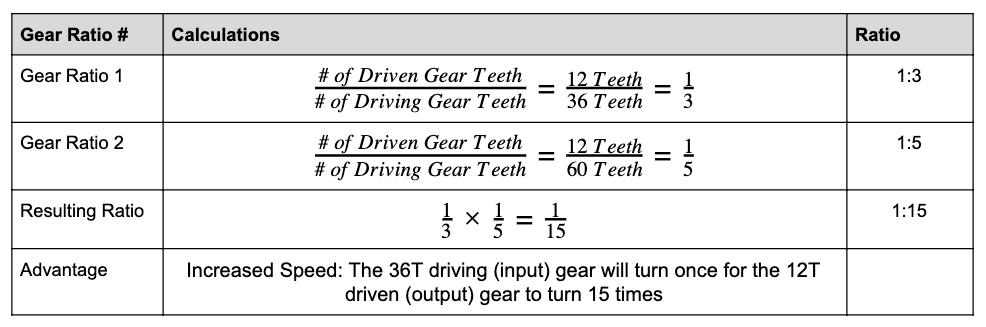In the example above, the Resulting Ratio row refers to calculating the Compound Gear Ratio by multiplying all of the individual gear ratios together.

Gear Ratio 1 has a 36 tooth-gear (36T gear) driving a 12 tooth-gear (12T gear). Viewing the relationship is Driven over Driving results in 12 over 36, which reduces down to one third. Thus, the ratio is 1:3.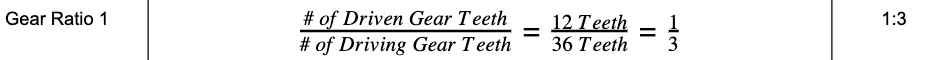Similarly for Gear Ratio 2, a 60T gear is driving a 12T gear. Viewing the relationship as Driven over Driving results in 12 over 60, which reduces to one fifth. Thus, the ratio is 1:5.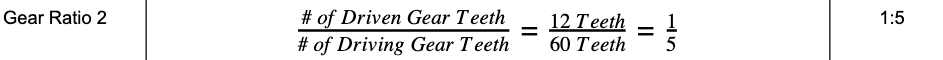To combine these two ratios, fraction multiplication is introduced. One third times one fifth is one fifteenth. Keep in mind, when multiplying fractions, you multiply straight across in the numerator and denominator. Thus, the compound gear ratio is 1:15.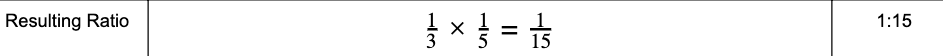Once the compounded gear ratio is calculated, it can now be determined what the mechanical advantage is. The resulting advantage is Increased Speed: The 36T driving (input) gear will turn once for the 12T driven (output) gear to turn 15 times.### Calculation 1

Fill in the missing calculations from the Gear Ratio table. Keep in mind, each person should be calculating according to their role.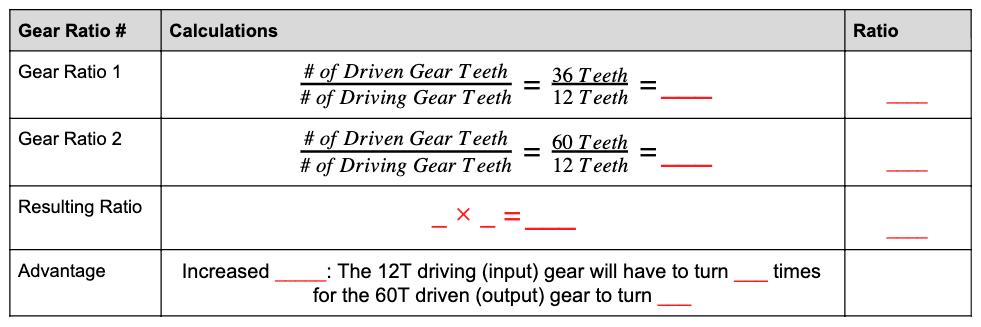• Role 1: Calculate the Gear Ratio 1 row of the above table. Show all work in your engineering notebook.

• Role 2: Calculate the Gear Ratio 2 row of the above table. Show all work in your engineering notebook.

• Role 3: Calculate the Resulting Ratio row of the above table. Check the calculations from Gear Ratio 1 and 2 before calculating the final compound gear ratio. Show all work in your engineering notebook.

• Role 4: Calculate the Advantage row of the above table. Show all work in your engineering notebook.

• All Roles: Once the table is completed, verify with all group members that the calculations are correct.Teacher Toolbox - Solution

Provided is the following solution table for Calculation 1. Notice that Calculate 1 is the inverse to the first example provided. Instead of the compound gear ratio being 1:15, the compound gear ratio is 15:1. Point this pattern out to students when going over the solution.

Further, call out the mathematical reasoning for the difference between speed and torque to ensure the students grasp the concept, as this will be addressed further in Teacher Toolbox for Mechanical Advantage on the following page.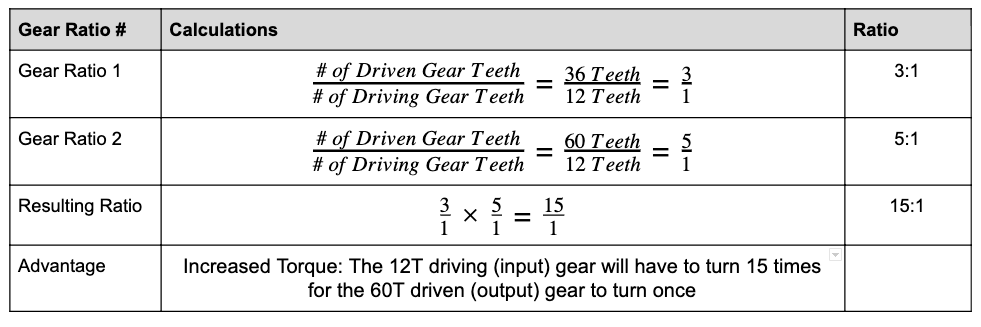Motivate Discussion

Q: What operation is used when calculating the compound gear ratio?
A: Multiplication. When multiplying fractions, multiply across the numerator and denominator.

Q: What does the mechanical advantage of increased torque mean for a robot in competition?
A: Answers may vary, but an example could include a robot lifting or carrying heavy objects.